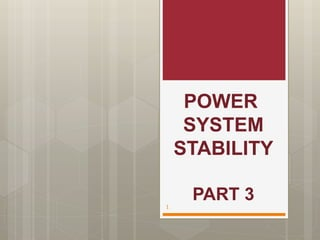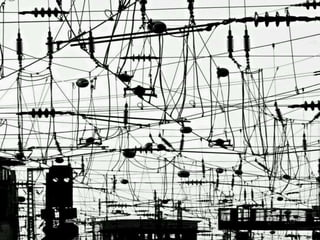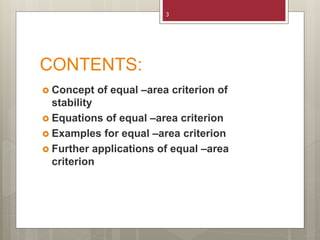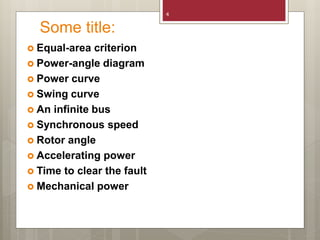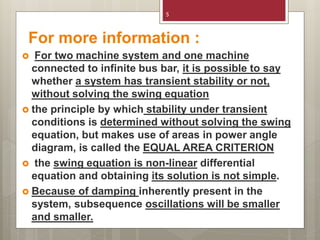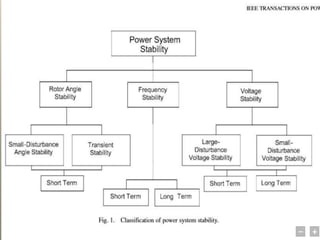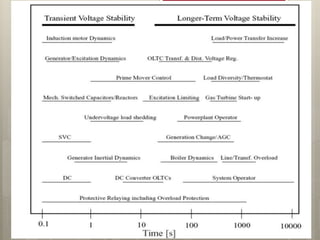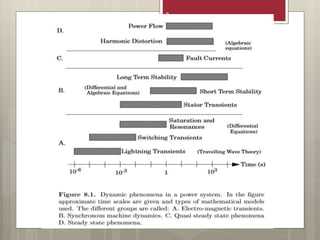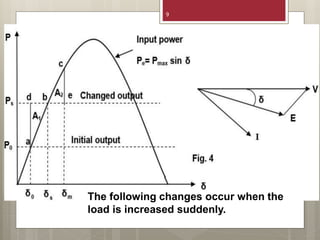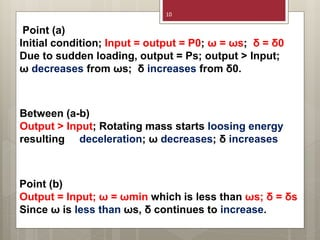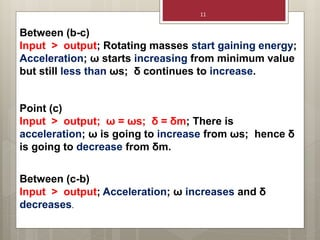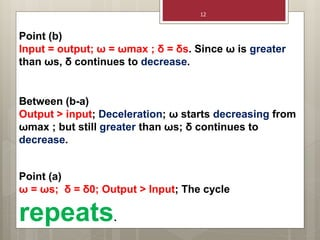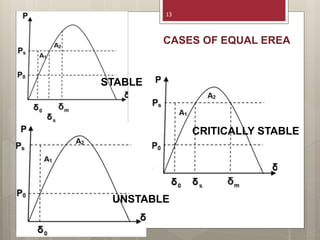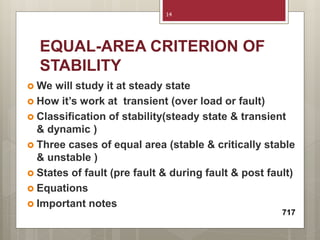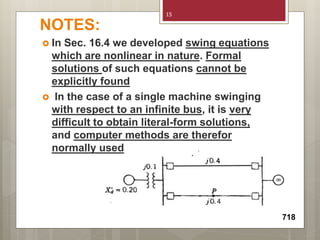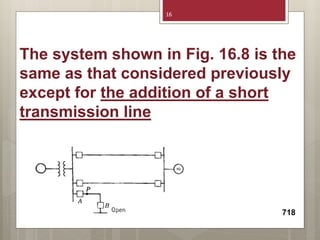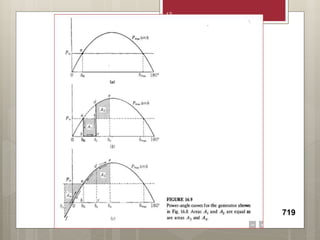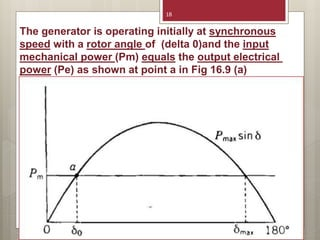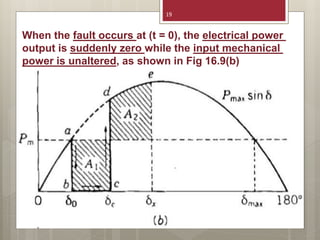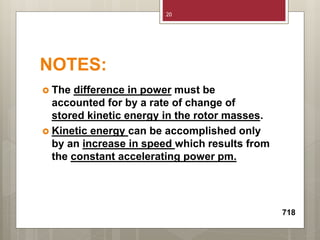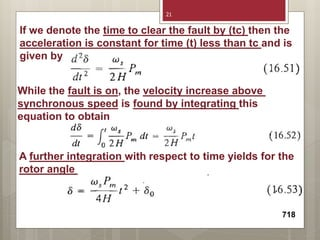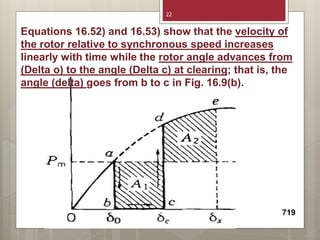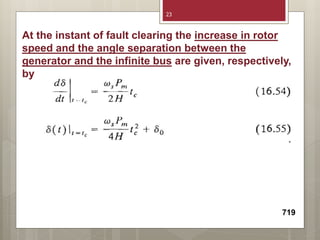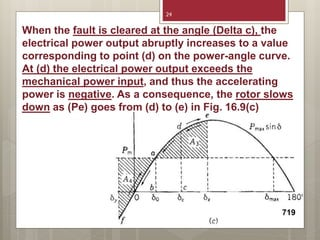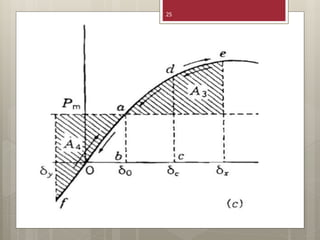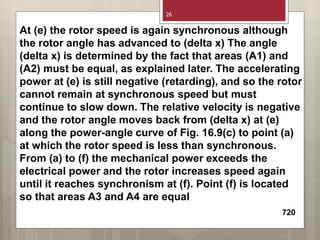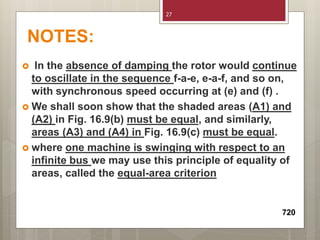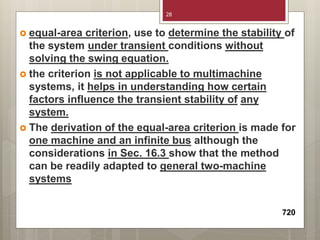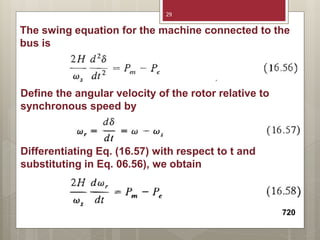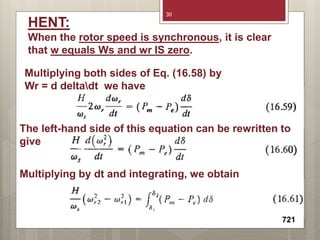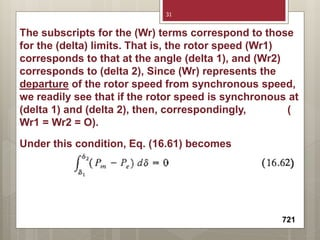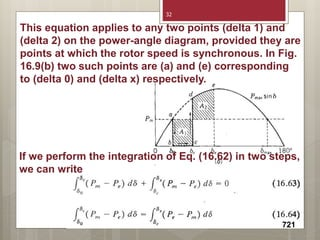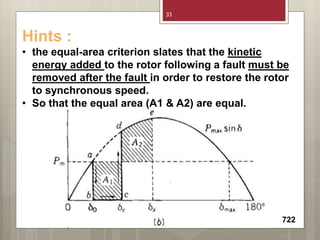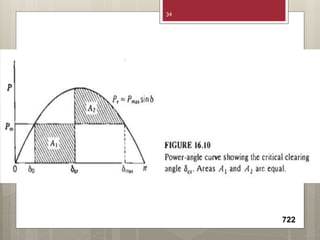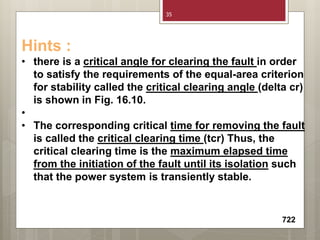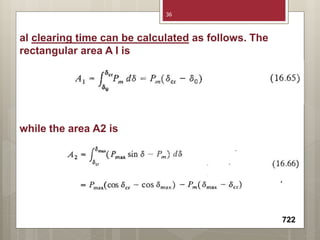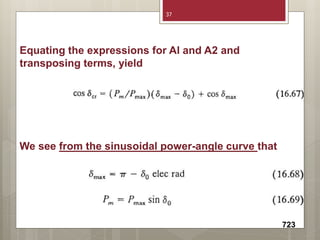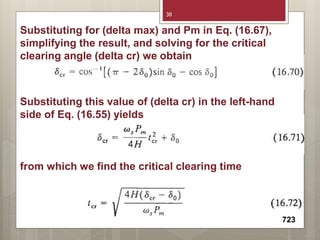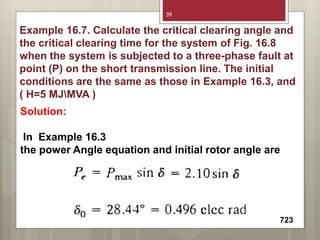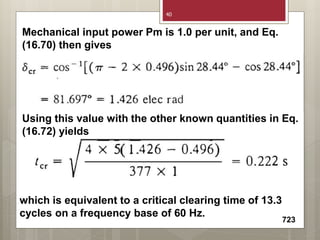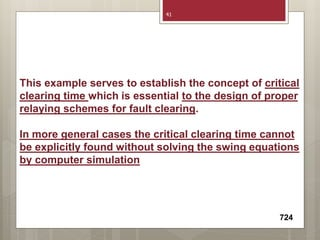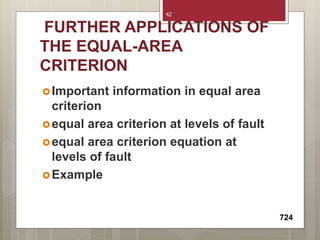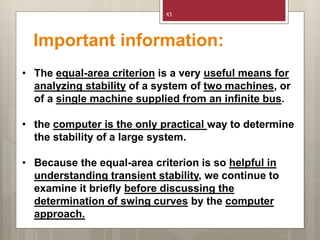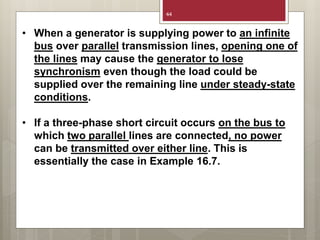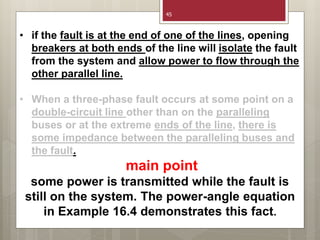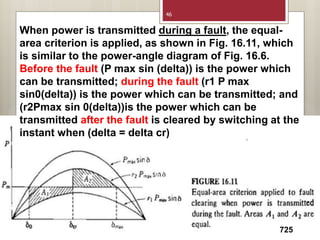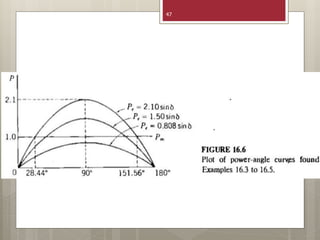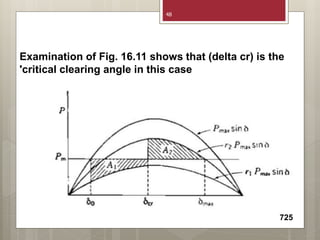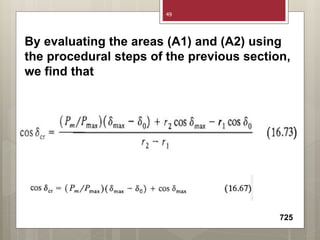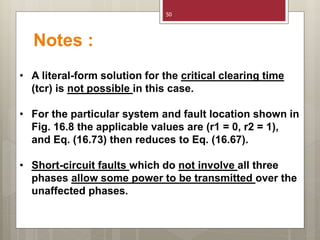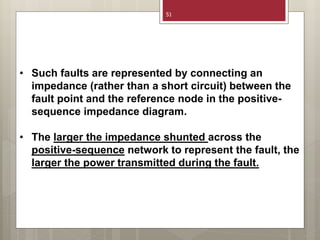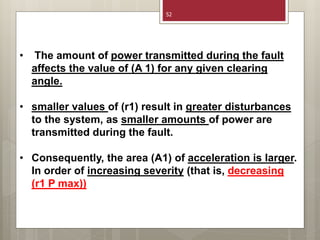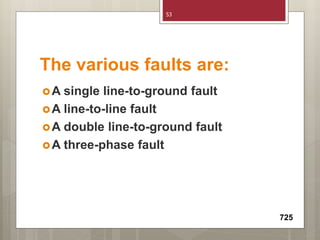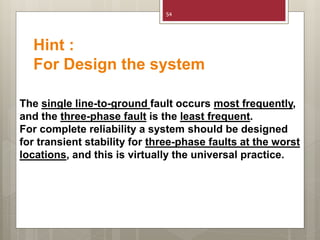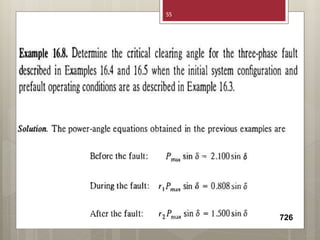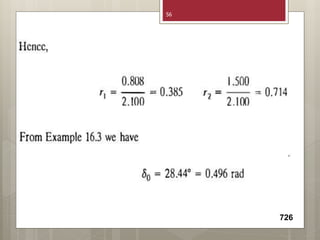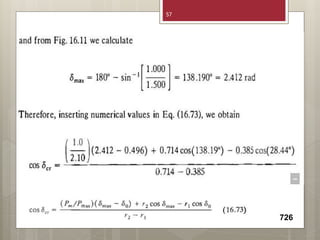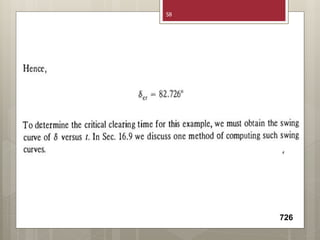1 de 59

### Power system stability 3.pptx

1. POWER SYSTEM STABILITY PART 3 1
2. 2
3. CONTENTS:  Concept of equal –area criterion of stability  Equations of equal –area criterion  Examples for equal –area criterion  Further applications of equal –area criterion 3
4. Some title:  Equal-area criterion  Power-angle diagram  Power curve  Swing curve  An infinite bus  Synchronous speed  Rotor angle  Accelerating power  Time to clear the fault  Mechanical power 4
5. For more information :  For two machine system and one machine connected to infinite bus bar, it is possible to say whether a system has transient stability or not, without solving the swing equation  the principle by which stability under transient conditions is determined without solving the swing equation, but makes use of areas in power angle diagram, is called the EQUAL AREA CRITERION  the swing equation is non-linear differential equation and obtaining its solution is not simple.  Because of damping inherently present in the system, subsequence oscillations will be smaller and smaller. 5
6. 6
7. 7
8. 8
9. 9 The following changes occur when the load is increased suddenly.
10. 10 Point (a) Initial condition; Input = output = P0; ω = ωs; δ = δ0 Due to sudden loading, output = Ps; output > Input; ω decreases from ωs; δ increases from δ0. Point (b) Output = Input; ω = ωmin which is less than ωs; δ = δs Since ω is less than ωs, δ continues to increase. Between (a-b) Output > Input; Rotating mass starts loosing energy resulting deceleration; ω decreases; δ increases
11. 11 Between (b-c) Input > output; Rotating masses start gaining energy; Acceleration; ω starts increasing from minimum value but still less than ωs; δ continues to increase. Point (c) Input > output; ω = ωs; δ = δm; There is acceleration; ω is going to increase from ωs; hence δ is going to decrease from δm. Between (c-b) Input > output; Acceleration; ω increases and δ decreases.
12. 12 Point (b) Input = output; ω = ωmax ; δ = δs. Since ω is greater than ωs, δ continues to decrease. Between (b-a) Output > input; Deceleration; ω starts decreasing from ωmax ; but still greater than ωs; δ continues to decrease. Point (a) ω = ωs; δ = δ0; Output > Input; The cycle repeats.
13. 13 CRITICALLY STABLE UNSTABLE STABLE CASES OF EQUAL EREA
14. EQUAL-AREA CRITERION OF STABILITY  We will study it at steady state  How it’s work at transient (over load or fault)  Classification of stability(steady state & transient & dynamic )  Three cases of equal area (stable & critically stable & unstable )  States of fault (pre fault & during fault & post fault)  Equations  Important notes 717 14
15. NOTES:  In Sec. 16.4 we developed swing equations which are nonlinear in nature. Formal solutions of such equations cannot be explicitly found  In the case of a single machine swinging with respect to an infinite bus, it is very difficult to obtain literal-form solutions, and computer methods are therefor normally used 718 15
16. The system shown in Fig. 16.8 is the same as that considered previously except for the addition of a short transmission line 718 16
17. 719 17
18. The generator is operating initially at synchronous speed with a rotor angle of (delta 0)and the input mechanical power (Pm) equals the output electrical power (Pe) as shown at point a in Fig 16.9 (a) 18
19. When the fault occurs at (t = 0), the electrical power output is suddenly zero while the input mechanical power is unaltered, as shown in Fig 16.9(b) 19
20. NOTES:  The difference in power must be accounted for by a rate of change of stored kinetic energy in the rotor masses.  Kinetic energy can be accomplished only by an increase in speed which results from the constant accelerating power pm. 718 20
21. If we denote the time to clear the fault by (tc) then the acceleration is constant for time (t) less than tc and is given by While the fault is on, the velocity increase above synchronous speed is found by integrating this equation to obtain A further integration with respect to time yields for the rotor angle 718 21
22. Equations 16.52) and 16.53) show that the velocity of the rotor relative to synchronous speed increases linearly with time while the rotor angle advances from (Delta o) to the angle (Delta c) at clearing; that is, the angle (delta) goes from b to c in Fig. 16.9(b). 719 22
23. At the instant of fault clearing the increase in rotor speed and the angle separation between the generator and the infinite bus are given, respectively, by 719 23
24. When the fault is cleared at the angle (Delta c), the electrical power output abruptly increases to a value corresponding to point (d) on the power-angle curve. At (d) the electrical power output exceeds the mechanical power input, and thus the accelerating power is negative. As a consequence, the rotor slows down as (Pe) goes from (d) to (e) in Fig. 16.9(c) 719 24
25. 25
26. At (e) the rotor speed is again synchronous although the rotor angle has advanced to (delta x) The angle (delta x) is determined by the fact that areas (A1) and (A2) must be equal, as explained later. The accelerating power at (e) is still negative (retarding), and so the rotor cannot remain at synchronous speed but must continue to slow down. The relative velocity is negative and the rotor angle moves back from (delta x) at (e) along the power-angle curve of Fig. 16.9(c) to point (a) at which the rotor speed is less than synchronous. From (a) to (f) the mechanical power exceeds the electrical power and the rotor increases speed again until it reaches synchronism at (f). Point (f) is located so that areas A3 and A4 are equal 720 26
27. NOTES:  In the absence of damping the rotor would continue to oscillate in the sequence f-a-e, e-a-f, and so on, with synchronous speed occurring at (e) and (f) .  We shall soon show that the shaded areas (A1) and (A2) in Fig. 16.9(b) must be equal, and similarly, areas (A3) and (A4) in Fig. 16.9(c) must be equal.  where one machine is swinging with respect to an infinite bus we may use this principle of equality of areas, called the equal-area criterion 720 27
28.  equal-area criterion, use to determine the stability of the system under transient conditions without solving the swing equation.  the criterion is not applicable to multimachine systems, it helps in understanding how certain factors influence the transient stability of any system.  The derivation of the equal-area criterion is made for one machine and an infinite bus although the considerations in Sec. 16.3 show that the method can be readily adapted to general two-machine systems 720 28
29. The swing equation for the machine connected to the bus is Define the angular velocity of the rotor relative to synchronous speed by Differentiating Eq. (16.57) with respect to t and substituting in Eq. 06.56), we obtain 720 29
30. HENT: When the rotor speed is synchronous, it is clear that w equals Ws and wr IS zero. Multiplying both sides of Eq. (16.58) by Wr = d deltadt we have The left-hand side of this equation can be rewritten to give Multiplying by dt and integrating, we obtain 721 30
31. The subscripts for the (Wr) terms correspond to those for the (delta) limits. That is, the rotor speed (Wr1) corresponds to that at the angle (delta 1), and (Wr2) corresponds to (delta 2), Since (Wr) represents the departure of the rotor speed from synchronous speed, we readily see that if the rotor speed is synchronous at (delta 1) and (delta 2), then, correspondingly, ( Wr1 = Wr2 = O). Under this condition, Eq. (16.61) becomes 721 31
32. This equation applies to any two points (delta 1) and (delta 2) on the power-angle diagram, provided they are points at which the rotor speed is synchronous. In Fig. 16.9(b) two such points are (a) and (e) corresponding to (delta 0) and (delta x) respectively. If we perform the integration of Eq. (16.62) in two steps, we can write 721 32
33. 722 Hints : • the equal-area criterion slates that the kinetic energy added to the rotor following a fault must be removed after the fault in order to restore the rotor to synchronous speed. • So that the equal area (A1 & A2) are equal. 33
34. 722 34
35. Hints : • there is a critical angle for clearing the fault in order to satisfy the requirements of the equal-area criterion for stability called the critical clearing angle (delta cr) is shown in Fig. 16.10. • • The corresponding critical time for removing the fault is called the critical clearing time (tcr) Thus, the critical clearing time is the maximum elapsed time from the initiation of the fault until its isolation such that the power system is transiently stable. 722 35
36. al clearing time can be calculated as follows. The rectangular area A I is 722 while the area A2 is 36
37. Equating the expressions for Al and A2 and transposing terms, yield We see from the sinusoidal power-angle curve that 723 37
38. from which we find the critical clearing time Substituting this value of (delta cr) in the left-hand side of Eq. (16.55) yields Substituting for (delta max) and Pm in Eq. (16.67), simplifying the result, and solving for the critical clearing angle (delta cr) we obtain 723 38
39. Solution: In Example 16.3 the power Angle equation and initial rotor angle are Example 16.7. Calculate the critical clearing angle and the critical clearing time for the system of Fig. 16.8 when the system is subjected to a three-phase fault at point (P) on the short transmission line. The initial conditions are the same as those in Example 16.3, and ( H=5 MJMVA ) 723 39
40. Using this value with the other known quantities in Eq. (16.72) yields Mechanical input power Pm is 1.0 per unit, and Eq. (16.70) then gives which is equivalent to a critical clearing time of 13.3 cycles on a frequency base of 60 Hz. 723 40
41. This example serves to establish the concept of critical clearing time which is essential to the design of proper relaying schemes for fault clearing. In more general cases the critical clearing time cannot be explicitly found without solving the swing equations by computer simulation 724 41
42. FURTHER APPLICATIONS OF THE EQUAL-AREA CRITERION Important information in equal area criterion equal area criterion at levels of fault equal area criterion equation at levels of fault Example 724 42
43. 43 • The equal-area criterion is a very useful means for analyzing stability of a system of two machines, or of a single machine supplied from an infinite bus. • the computer is the only practical way to determine the stability of a large system. • Because the equal-area criterion is so helpful in understanding transient stability, we continue to examine it briefly before discussing the determination of swing curves by the computer approach. Important information:
44. 44 • When a generator is supplying power to an infinite bus over parallel transmission lines, opening one of the lines may cause the generator to lose synchronism even though the load could be supplied over the remaining line under steady-state conditions. • If a three-phase short circuit occurs on the bus to which two parallel lines are connected, no power can be transmitted over either line. This is essentially the case in Example 16.7.
45. 45 • if the fault is at the end of one of the lines, opening breakers at both ends of the line will isolate the fault from the system and allow power to flow through the other parallel line. • When a three-phase fault occurs at some point on a double-circuit line other than on the paralleling buses or at the extreme ends of the line, there is some impedance between the paralleling buses and the fault. main point some power is transmitted while the fault is still on the system. The power-angle equation in Example 16.4 demonstrates this fact.
46. 725 46 When power is transmitted during a fault, the equal- area criterion is applied, as shown in Fig. 16.11, which is similar to the power-angle diagram of Fig. 16.6. Before the fault (P max sin (delta)) is the power which can be transmitted; during the fault (r1 P max sin0(delta)) is the power which can be transmitted; and (r2Pmax sin 0(delta))is the power which can be transmitted after the fault is cleared by switching at the instant when (delta = delta cr)
47. 47
48. 725 48 Examination of Fig. 16.11 shows that (delta cr) is the 'critical clearing angle in this case
49. By evaluating the areas (A1) and (A2) using the procedural steps of the previous section, we find that 725 49
50. 50 • A literal-form solution for the critical clearing time (tcr) is not possible in this case. • For the particular system and fault location shown in Fig. 16.8 the applicable values are (r1 = 0, r2 = 1), and Eq. (16.73) then reduces to Eq. (16.67). • Short-circuit faults which do not involve all three phases allow some power to be transmitted over the unaffected phases. Notes :
51. 51 • Such faults are represented by connecting an impedance (rather than a short circuit) between the fault point and the reference node in the positive- sequence impedance diagram. • The larger the impedance shunted across the positive-sequence network to represent the fault, the larger the power transmitted during the fault.
52. 52 • The amount of power transmitted during the fault affects the value of (A 1) for any given clearing angle. • smaller values of (r1) result in greater disturbances to the system, as smaller amounts of power are transmitted during the fault. • Consequently, the area (A1) of acceleration is larger. In order of increasing severity (that is, decreasing (r1 P max))
53. The various faults are: A single line-to-ground fault A line-to-line fault A double line-to-ground fault A three-phase fault 725 53
54. 54 The single line-to-ground fault occurs most frequently, and the three-phase fault is the least frequent. For complete reliability a system should be designed for transient stability for three-phase faults at the worst locations, and this is virtually the universal practice. Hint : For Design the system
55. 726 55
56. 726 56
57. 726 57
58. 726 58
59. THANKS 59